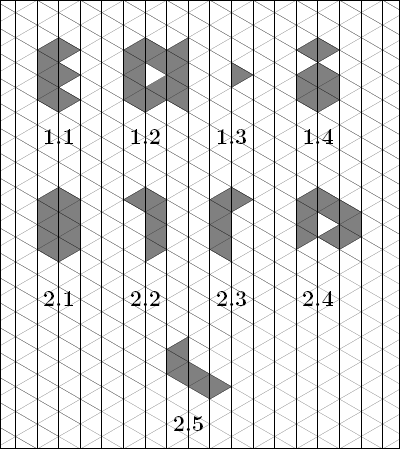시간 제한메모리 제한제출정답맞힌 사람정답 비율
1 초 128 MB0000.000%

## 문제

A triangular grid consists in equilateral triangles with side length 1 (see p. 3). A path in the triangular grid is an arbitrary finite sequence of triangles (with side length 1) of the grid such that every two successive ones share a side.

A shape formed by the points of any finite number of triangles is called an isle if any two triangles of the grid contained in this shape are connected by some path formed by the triangles contained in the shape.The shapes in the figures 1.1, 1.2 and 1.3 are isles. The shape in the figure 1.4 is not an isle. The shapes in the figures 2.2, 2.3 and 2.5 are congruent.

We aim at obtaining a systematic description, for each n ≤ 10, of all non-congruent isles that can be formed by n triangles with side length 1, and calculating how many such isles there are.

The boundary of every isle formed by at most ten triangles is a polygonal chain consisting of unitary segments of the grid. It can be revolved about, i.e. it can be contoured without detaching pencil from the sheet, in such a way that its every segment is followed once, and we come back to the initial point. It may happen though that some point has to be crossed more than once (see Figure 2.4).

Luckily, in case of isles formed by at most ten triangles the shape's perimeter is connected (and can be thus contoured without detaching pencil from the sheet), unlike the one in Figure 1.2.

While circling around the perimeter, after each unit segment we make a turn of either of the following types:

• a - 120 degrees left,
• b - 60 degrees left,
• c - 0 degrees (i.e. no turn, actually),
• d - 60 degrees right,
• e - 120 degrees right.

Each cycle around the isle can be described by a word consisting of letters from the set {a,b,c,d,e}, where each letter tells which turn should be made after each successive unit segment the perimeter consists of. The cycle description has as many letters as there are such unit segments. This means we also describe the turn after the last segment of the polygonal chain, even though it is not required to uniquely determine the shape. This redundant letter is, however, very helpful in transforming one description of a cycle around the shape to another one that differs only in the initial point.
The words cdddcddd, dcdddcdd, cbbbcbbb describe different cycles around the shape of the Figure 2.1.

The words cbeddcde, adcabcbb, abcbbadc describe different cycles around the shape of the Figure 2.2.

The words acdabbcb i cddebced describe different cycles around the shape of the Figure 2.3.

If the inside of the shape is constantly on right hand side during a cycle around some shape, we call such cycle a clockwise cycle.

One can determine, for each isle, the set of all isles congruent with it and these isles' clockwise cycles. The code of an isle is such a word that:

1. it is a description of a clockwise cycle around the contour of some isle congruent with the latter,
2. it is the lexicographically smallest of all words satisfying the previous condition.

For the isle depicted in Figures 2.2 and 2.3, which are congruent, we take into account all clockwise cycles around each of them:

beddcdec, eddcdecb, ddcdecbe, dcdecbed, cdecbedd, decbeddc, ecbeddcd, cbeddcde

and

bcedcdde, cedcddeb, edcddebc, dcddebce, cddebced, ddebcedc, debcedcd, ebcedcdd

so their common code is: bcedcdde, the lexicographically smallest of all the words above.

The code of the isle depicted in Figure 2.4 is: aadecddcddde.

Write a programme that:

• for a given code of an isle of size k generates the codes of all isles of size k+1 that can be obtained from the latter by adding one triangle to it,
• for a given integer n generates the codes of all isles of size n.

## 입력

In the first line of the standard input an integer t (1 ≤ t ≤ 5), denoting the number of queries, is given. Each of the following t lines contains a query of some type. The query of type 1 consists of the letter K and a code of an isle formed by at most ten triangles, separated by a single space. The query of type 2 consists of the letter N and an integer n (1 ≤ n ≤ 10), separated by a single space.

## 출력

The answers to the queries should be printed out to the standard output.

For queries of type 1 the number of distinct codes of isles that can be obtained by adding one triangle from isles congruent to the one described by the given code. In the following line all these codes, separated by single spaces, should be printed in lexicographic order.

For queries of type 2 the number of distinct codes of isles formed by n triangles should be printed. In the following line all these codes should be printed in lexicographic order.

## 예제 입력 1

2

5